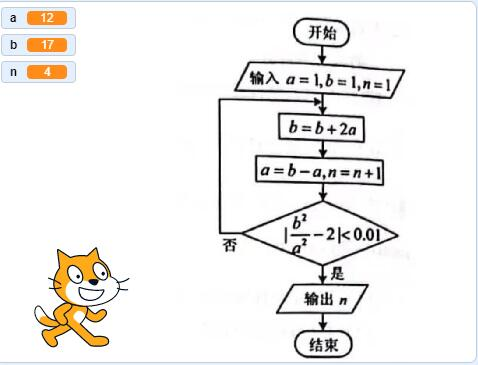2023-05-20
1012```#第一步，定义变量并赋值
a,b,n=1,1,1
#第二步
b=b+2*a
#第三步
a=b-a
n=n+1
#第四步
while abs(b**2/a**2-2)>=0.01:#不小于就是大于等于
print(abs(b**2/a**2-2))
b=b+2*a
a=b-a
n=n+1
print(n)```

```#include#include

using namespace std;
int main()
{
double a,b,n;
a=b=n=1;
b=b+2*a;
a=b-a;
n=n+1;
while(abs(pow(b,2)/pow(a,2)-2)>=0.01){
b=b+2*a;
a=b-a;
n=n+1;
}
cout << n;
return 0;
}
```

```public class Test {
public static void main(String []args) {
double a,b,n;
a=b=n=1;
b=b+2*a;
a=b-a;
n=n+1;
while(Math.abs(Math.pow(b, 2)/Math.pow(a, 2)-2)>=0.01){
b=b+2*a;
a=b-a;
n=n+1;
}
System.out.println(n);
}
}```

```\$a=\$b=\$n=1;
\$b=\$b+2*\$a;
\$a=\$b-\$a;
\$n=\$n+1;
while(abs(pow(\$b, 2)/pow(\$a, 2)-2)>=0.01){
\$b=\$b+2*\$a;
\$a=\$b-\$a;
\$n=\$n+1;
}
echo \$n;```

### 本期高人气挑战队员﻿